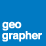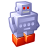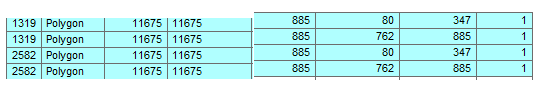# Output name to be the same as the input name (in a new folder) after processing

4057
2
09-01-2015 07:42 AMby
New Contributor II

Hi,

I was following this simple example from ESRI (ArcGIS Desktop 😞

import arcpy

from arcpy import env

import os

# The workspace environment needs to be set before ListFeatureClasses

# to identify which workspace the list will be based on

env.workspace = "c:/data"

out_workspace = "c:/data/results/"

clip_features = "c:/data/testarea/boundary.shp"

# Loop through a list of feature classes in the workspace

for fc in arcpy.ListFeatureClasses():

# Set the output name to be the same as the input name, and

# locate in the 'out_workspace' workspace

#

output = os.path.join(out_workspace, fc)

# Clip each input feature class in the list

#

arcpy.Clip_analysis(fc, clip_features, output, 0.1)

I would like to run a very similar code with "Identity_analysis", however, I receive an error message when run my code, as it wants to create only one shapefile rather many with the same name(s)  as the input file(s). Here is the code. It generates only one file " identity_country.shp" and than gives me an error msg that the file already exist.

import arcpy

from arcpy import env

import os

dirpath = r'C:\reggie\stat\spatial'

arcpy.env.workspace = dirpath

#set local parameters

identity_feature = r'C:\reggie\stat\GIS_stats\country.shp'

out_workspace = r'C:\reggie\stat\spatial\identity_country'

for fc in arcpy.ListFeatureClasses("*"):

output = os.path.join(out_workspace, fc)

arcpy.Identity_analysis(fc, identity_feature, output, "ALL", "", "NO_RELATIONSHIPS")

Tags (3)
1 Solution

Accepted SolutionsOccasional Contributor III

Does your output shapefile already exists in your output folder? If yes, then you would need to set the overwrite property to True or use python logic to check if the output shapefile exists and delete it if does prior to calling the tool that will generate it. I've shown two options for this below.

```# ## OPTION A ## #
import arcpy
from os import path
arcpy.env.overwriteOutput = True
arcpy.env.workspace = r"C:\reggie\stats\spatial"
idFeat = r""
outWS  = r""
for fc in arcpy.ListFeatureClasses():
output = path.join(outWS, fc)
arcpy.analysis.Identity(fc, idFeat, output, relationship="NO_RELATIONSHIPS")

# ## OPTION B ## #
import arcpy
from os import path
arcpy.env.workspace = r"C:\reggie\stats\spatial"
idFeat = r""
outWS  = r""
for fc in arcpy.ListFeatureClasses():
output = path.join(outWS, fc)
if arcpy.Exists(output):
arcpy.management.Delete(output)
arcpy.analysis.Identity(fc, idFeat, output, relationship="NO_RELATIONSHIPS")    ```
2 RepliesOccasional Contributor III

Does your output shapefile already exists in your output folder? If yes, then you would need to set the overwrite property to True or use python logic to check if the output shapefile exists and delete it if does prior to calling the tool that will generate it. I've shown two options for this below.

```# ## OPTION A ## #
import arcpy
from os import path
arcpy.env.overwriteOutput = True
arcpy.env.workspace = r"C:\reggie\stats\spatial"
idFeat = r""
outWS  = r""
for fc in arcpy.ListFeatureClasses():
output = path.join(outWS, fc)
arcpy.analysis.Identity(fc, idFeat, output, relationship="NO_RELATIONSHIPS")

# ## OPTION B ## #
import arcpy
from os import path
arcpy.env.workspace = r"C:\reggie\stats\spatial"
idFeat = r""
outWS  = r""
for fc in arcpy.ListFeatureClasses():
output = path.join(outWS, fc)
if arcpy.Exists(output):
arcpy.management.Delete(output)
arcpy.analysis.Identity(fc, idFeat, output, relationship="NO_RELATIONSHIPS")    ```by
New Contributor II

Hi Freddie,

Both worked like charm, million thanks!

Additional Q - as the input vector contains many polygons while the identify feature has only one polygon, however, when the identify feature crosses the input feature I receive two or more readings (with the same FID) . See it below:Could I set up the code in a way, that the input polygon is either and only in or out of the identify feature but not in both?